# Precalculus : Graph Exponential Functions

## Example Questions

### Example Question #1 : Graph Exponential Functions

Choose the description below that matches the equation: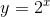Exponential growth

Y-intercept at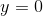Exponential decay

Y-intercept at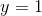Exponential growth

Y-intercept atExponential decay

Y-intercept atExponential growth

Y-intercept at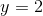Exponential growth

Y-intercept atExplanation:

Exponential graphs can either decay or grow. This is based on the value of the base of the exponent. If the base is greater than, the graph will be growth. And, if the base is less than, then the graph will be decay. In this situation, our base is. Since this is greater than, we have a growth graph. Then, to determine the y-intercept we substitute. Thus, we get: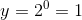for the y-intercept.

### Example Question #2 : Graph Exponential Functions

Choose the description that matches the equation below: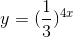Exponential growth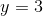Exponential decayExponential decay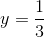Exponential decay

Y-interceptExponential growthExponential decay

Y-interceptExplanation:

Exponential graphs can either decay or grow. This is based on the value of the base of the exponent. If the base is greater than, the graph will be growth. And, if the base is less than, then the graph will be decay. In this situation, our base is. Since this is less than, we have a decay graph. Then, to determine the y-intercept we substitute. Thus, we get: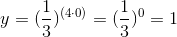for the y-intercept.

### All Precalculus Resources